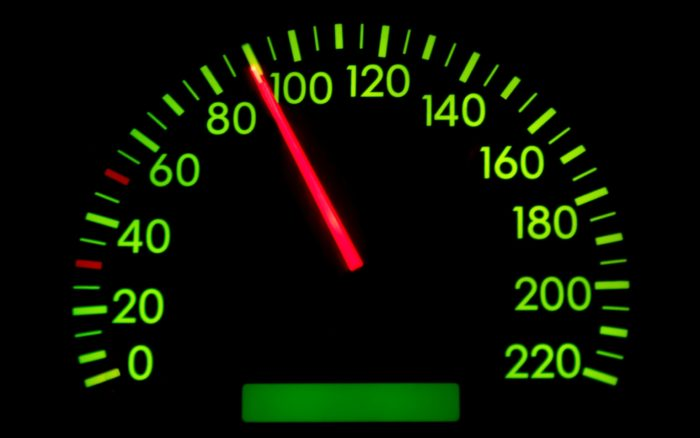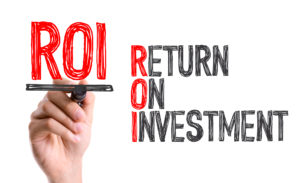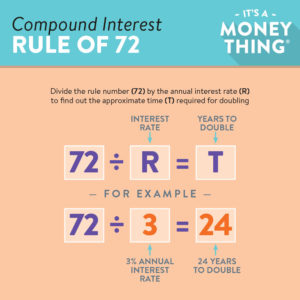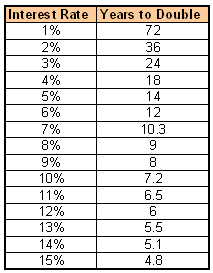The Speedometer of Trading: Return On Investment (ROI) and The Rule of 72

An experienced trader in selling commodity options or any other investment should know her ROI, Return On Investment.  This is the primary way to know “the bang for the buck” an investor is achieving.  For example, if I sell a Natural Gas CALL option with a strike of 6.0 and my initial margin requirement is \$330 for an investment that may return \$90. Here’s how to compute the ROI, return on investment:  Yield = \$90  Margin Requirement = \$330, then 90/330 = 27% in the time until the option expires which is only 88 days from date the trade was placed on NOV 27, 2017.  So the annualized return = (365/88) * 27% = 112%.I am not implying that you can expect to make a return of 112% annually on your entire account; that would require you to remain 100% invested at all times.  When trading short commodity options, it is quite common to be only 50% and often less on the average – so you always have plenty of room for possible margin requirements.

The ROI of commodity options is far higher than that of selling options on stocks due to the high leverage of very low commodity margin requirements (“SPAN minimums”).  Far OTM (Out-of-the-Money) commodity options with very low deltas require relatively small amounts of margin to place trades.  The result is very high ROI, and a lot of “bang for the buck” as the saying goes.  Often the ROI of selling options on commodities is 5 to 10 times that of trading stock options in the same way.

Here’s a quick way to see how long it takes money to double at any given interest rate.  This is called THE RULE OF 72.

Dividing 72 by your annual rate = the number of years it takes for your investment to double.
Examples:
72 divided by 6% = You money doubles every 12 years.
72 divided by 15% = Your money doubles every 4.8 years.

Dividing 72 by your annual yield % = the number of years it takes to double your money:
Examples:
72 divided by 10% return = Your money will double in 7.2 years.
72 divided by 3% (CD rate) = Your money will double in 24 years.Here’s an article on how to avoid large losses in your selling commodity options account: Account Management